### Study on bias and interpretable models - SCHOOL PROJECT¶

Nathan Lauga - December 2018

Hello world ! ✋

## 1. Load and prepare data¶

In :
%matplotlib inline
# data manipulation libraries
import pandas as pd
import numpy as np

from time import time

# Graphs libraries
import matplotlib
import matplotlib.pyplot as plt
import matplotlib.patches as patches
plt.style.use('seaborn-white')
import seaborn as sns

import plotly.offline as py
py.init_notebook_mode(connected=True)
import plotly.graph_objs as go
import plotly.tools as tls
import plotly.figure_factory as ff
from plotly import tools

# Custom visualisation functions in visualitions.py file
from visualisations import plot_fair_metrics, plot_bar, plot_histo, plot_model_performance,\
plot_fair_metrics_plotly, plot_score_fair_metrics, plot_lime_importance,\
plot_compare_model_performance, plot_global_importance_lime

# Custom metrics functions in metrics.py file
from metrics import fair_metrics, get_model_performance, score_fair_metrics, score_all_attr

# Libraries to study
from aif360.datasets import StandardDataset
from aif360.metrics import BinaryLabelDatasetMetric, ClassificationMetric
from aif360.algorithms.preprocessing import LFR, Reweighing
from aif360.algorithms.postprocessing import CalibratedEqOddsPostprocessing, EqOddsPostprocessing, RejectOptionClassification

import lime
import lime.lime_tabular
import shap

# ML libraries
from sklearn.preprocessing import MinMaxScaler, LabelEncoder
from sklearn.ensemble import RandomForestClassifier
import tensorflow as tf

# Design libraries
from IPython.display import Markdown, display
import warnings
warnings.filterwarnings("ignore")


I choose to use the Adult dataset that you can find on the following link : UCI Machine Learning Repository : Adult dataset

In :
cols = ["Age", "Workclass", "Final Weight", "Education", "Education-Num", "Marital Status","Occupation", "Relationship", "Race", "Sex", "Capital Gain", "Capital Loss","Hours per week", "Country", "Income"]
data.columns = cols


### 1.3 Quick EDA¶

In :
data.head()

Out:
Age Workclass Final Weight Education Education-Num Marital Status Occupation Relationship Race Sex Capital Gain Capital Loss Hours per week Country Income
0 39 State-gov 77516 Bachelors 13 Never-married Adm-clerical Not-in-family White Male 2174 0 40 United-States <=50K
1 50 Self-emp-not-inc 83311 Bachelors 13 Married-civ-spouse Exec-managerial Husband White Male 0 0 13 United-States <=50K
2 38 Private 215646 HS-grad 9 Divorced Handlers-cleaners Not-in-family White Male 0 0 40 United-States <=50K
3 53 Private 234721 11th 7 Married-civ-spouse Handlers-cleaners Husband Black Male 0 0 40 United-States <=50K
4 28 Private 338409 Bachelors 13 Married-civ-spouse Prof-specialty Wife Black Female 0 0 40 Cuba <=50K

Since Education-Num is the same as Education but classed by number we can drop it

In :
data.drop(columns=['Education-Num'], inplace=True)

In :
na_count = pd.DataFrame(data.apply(lambda x : x.isna()).sum()).transpose()
na_count

Out:
Age Workclass Final Weight Education Marital Status Occupation Relationship Race Sex Capital Gain Capital Loss Hours per week Country Income
0 0 0 0 0 0 0 0 0 0 0 0 0 0 0

This dataset doesn't have any NA value so we don't have to work with that.

In :
# print(data.columns.values)
display(Markdown('#### Continuous columns'))
plot_histo(data, 'Age')
plot_histo(data, 'Final Weight')
plot_histo(data, 'Capital Gain', type='log')
plot_histo(data, 'Capital Loss', type='log')
plot_histo(data, 'Hours per week')


#### Continuous columns¶

In :
display(Markdown('#### Categorical columns'))
plot_bar(data, 'Workclass')
plot_bar(data, 'Education')
plot_bar(data, 'Marital Status')
plot_bar(data, 'Relationship')
plot_bar(data, 'Race')
plot_bar(data, 'Sex')
plot_bar(data, 'Country')
plot_bar(data, 'Income')


### 1.4 Work with feature¶

#### Encoding categorical columns¶

Why encoding and not dummies ?

Later in the notebook I will use lime and shap libraries to interpret the model but with dummies values it just get the data to be incoherent (I will explain it later).

In :
categorical_features = ["Workclass", "Education", "Marital Status","Occupation", "Relationship", "Race", "Sex", "Country", "Income"]
categorical_features_idx = [np.where(data.columns.values == col) for col in categorical_features]

In :
data_encoded = data.copy()

categorical_names = {}
encoders = {}

# Use Label Encoder for categorical columns (including target column)
for feature in categorical_features:
le = LabelEncoder()
le.fit(data_encoded[feature])

data_encoded[feature] = le.transform(data_encoded[feature])

categorical_names[feature] = le.classes_
encoders[feature] = le


#### Scale numerical columns¶

I choose to scale these columns because later in this notebook I will use a neural network so it's necessary to use it.

In :
numerical_features = [c for c in data.columns.values if c not in categorical_features]

for feature in numerical_features:
val = data_encoded[feature].values[:, np.newaxis]
mms = MinMaxScaler().fit(val)
data_encoded[feature] = mms.transform(val)
encoders[feature] = mms

data_encoded = data_encoded.astype(float)

In :
data_encoded.head()

Out:
Age Workclass Final Weight Education Marital Status Occupation Relationship Race Sex Capital Gain Capital Loss Hours per week Country Income
0 0.301370 7.0 0.044302 9.0 4.0 1.0 1.0 4.0 1.0 0.02174 0.0 0.397959 39.0 0.0
1 0.452055 6.0 0.048238 9.0 2.0 4.0 0.0 4.0 1.0 0.00000 0.0 0.122449 39.0 0.0
2 0.287671 4.0 0.138113 11.0 0.0 6.0 1.0 4.0 1.0 0.00000 0.0 0.397959 39.0 0.0
3 0.493151 4.0 0.151068 1.0 2.0 6.0 0.0 2.0 1.0 0.00000 0.0 0.397959 39.0 0.0
4 0.150685 4.0 0.221488 9.0 2.0 10.0 5.0 2.0 0.0 0.00000 0.0 0.397959 5.0 0.0

## 2. AIF360 Introduction¶

### 2.1 Create dataset using aif360¶

In :
privileged_sex = np.where(categorical_names['Sex'] == ' Male')
privileged_race = np.where(categorical_names['Race'] == ' White')

In :
data_orig = StandardDataset(data_encoded,
label_name='Income',
favorable_classes=,
protected_attribute_names=['Sex', 'Race'],
privileged_classes=[privileged_sex, privileged_race])


### 2.2 Quick look on the dataset¶

In :
# print out some labels, names, etc.
display(Markdown("#### Dataset shape"))
print(data_orig.features.shape)
display(Markdown("#### Favorable and unfavorable labels"))
print(data_orig.favorable_label, data_orig.unfavorable_label)
display(Markdown("#### Protected attribute names"))
print(data_orig.protected_attribute_names)
display(Markdown("#### Privileged and unprivileged protected attribute values"))
print(data_orig.privileged_protected_attributes, data_orig.unprivileged_protected_attributes)
display(Markdown("#### Dataset feature names"))
print(data_orig.feature_names)


#### Dataset shape¶

(32561, 13)


#### Favorable and unfavorable labels¶

1.0 0.0


#### Protected attribute names¶

['Sex', 'Race']


#### Privileged and unprivileged protected attribute values¶

[array([1.]), array([4.])] [array([0.]), array([0., 1., 2., 3.])]


#### Dataset feature names¶

['Workclass', 'Relationship', 'Final Weight', 'Sex', 'Age', 'Race', 'Country', 'Occupation', 'Hours per week', 'Education', 'Marital Status', 'Capital Loss', 'Capital Gain']


### 2.3 First model¶

#### Split into train and test set¶

In :
np.random.seed(42)

data_orig_train, data_orig_test = data_orig.split([0.7], shuffle=True)

display(Markdown("#### Train Dataset shape"))
print(data_orig_train.features.shape)
display(Markdown("#### Test Dataset shape"))
print(data_orig_test.features.shape)


#### Train Dataset shape¶

(22792, 13)


#### Test Dataset shape¶

(9769, 13)


#### Training the model : classic Random Forest¶

In :
def train_model(model, dataset):
X_train = dataset.features
y_train = dataset.labels.ravel()

# Train the model
model.fit(X_train, y_train, sample_weight=dataset.instance_weights)

return model

# Model to learn
rf = RandomForestClassifier(n_estimators=100)

# Train and save the model
rf_orig = train_model(rf, data_orig_train)


#### Predict on test set¶

In :
X_test = data_orig_test.features
y_test = data_orig_test.labels.ravel()


Now we have a model (Random forest) that is trained with the training dataset and the prediction of the test set. So in a first place let's check the performance of our model (accuracy, F1 Score, confusion matrix, ...).

#### Performance of the model¶

In :
plot_model_performance(rf_orig, data_orig_test.features, y_test)


#### Accuracy of the model :¶

0.8606817483877572


#### F1 score of the model :¶

0.6835619623343407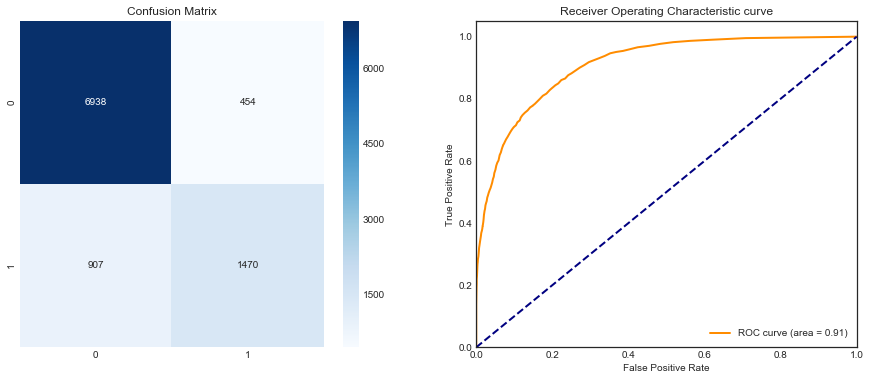## 3. Bias and Fairness¶

Today, a problem of the model that can be produce by Machine Learning is bias that data can have. So a question is how to measure those bias and how to avoid them. In python there is a package produced by IBM called aif360 that can gives us some metrics and algorithms to know if our data / model are bias and to get a fair model.

### 3.1 Metrics¶

So with aif360 we have some metrics that indicate if our data or model are bias. I will use 5 metrics :

• Statistical Parity Difference
• Equal Opportunity Difference
• Average Absolute Odds Difference
• Disparate Impact
• Theil Index

#### 3.1.1 Statistical Parity Difference¶

This measure is based on the following formula :

$$Pr(Y=1|D=unprivileged) - Pr(Y=1|D=privileged)$$

Here the bias or statistical imparity is the difference between the probability that a random individual drawn from unprivileged is labeled 1 (so here that he has more than 50K for income) and the probability that a random individual from privileged is labeled 1.

So it has to be close to 0 so it will be fair.

Also you can find more details about that here : One definition of algorithmic fairness: statistical parity

#### 3.1.2 Equal Opportunity Difference¶

This metric is just a difference between the true positive rate of unprivileged group and the true positive rate of privileged group so it follows this formula :

$$TPR_{D=unprivileged} - TPR_{D=privileged}$$

Same as the previous metric we need it to be close to 0.

#### 3.1.3 Average Absolute Odds Difference¶

This measure is using both false positive rate and true positive rate to calculate the bias. It's calculating the equality of odds with the next formula :

$$\frac{1}{2}[|FPR_{D=unprivileged} - FPR_{D=privileged} | + | TPR_{D=unprivileged} - TPR_{D=privileged}|]$$

It needs to be equal to 0 to be fair.

#### 3.1.4 Disparate Impact¶

For this metric we use the following formula :

$$\frac{Pr(Y=1|D=unprivileged)}{Pr(Y=1|D=privileged)}$$

Like the first metric we use both probabities of a random individual drawn from unprivileged or privileged with a label of 1 but here it's a ratio.

It changes the objective, for the disparate impact it's 1 that we need.

#### 3.1.5 Theil Index¶

This measure is also known as the generalized entropy index but with $\alpha$ equals to 1 (more informations on the Wikipedia page). So we can calculate it with this formula :

$$\frac{1}{n} \sum_{i=0}^{n} \frac{b_i}{\mu} ln \frac{b_i}{\mu}$$

Where $b_i = \hat{y}_i - y_i + 1$

So it needs to be close to 0 to be fair.

With these differents metrics we can see that for some we need the prediction and for others just the original dataset. This is why we will use 2 classes of the aif360 package : ClassificationMetric and BinaryLabelDatasetMetric.

For the first one we need the prediction so we can have the following metrics :

equal_opportunity_difference()

average_abs_odds_difference()

theil_index()

Then for the metrics that don't require prediction (the second class) we can use them with the following functions :

statistical_parity_difference()

disparate_impact()

Now I construct a DataFrame that will keep the values of the differents metrics I talked just above with a function.

In :
def get_fair_metrics_and_plot(data, model, plot=True, model_aif=False):
pred = model.predict(data).labels if model_aif else model.predict(data.features)
# fair_metrics function available in the metrics.py file
fair = fair_metrics(data, pred)

if plot:
# plot_fair_metrics function available in the visualisations.py file
# The visualisation of this function is inspired by the dashboard on the demo of IBM aif360
plot_fair_metrics(fair)
display(fair)

return fair

In :
fair = get_fair_metrics_and_plot(data_orig_test, rf_orig)

WARNING:root:There are some instances in the dataset which are not designated as either privileged or unprivileged. Are you sure this is right?
WARNING:root:There are some instances in the dataset which are not designated as either privileged or unprivileged. Are you sure this is right?


### Check bias metrics :¶

A model can be considered bias if just one of these five metrics show that this model is biased.

#### For the Sex attribute :¶

With default thresholds, bias against unprivileged group detected in 3 out of 5 metrics

#### For the Race attribute :¶

With default thresholds, bias against unprivileged group detected in 4 out of 5 metrics

statistical_parity_difference equal_opportunity_difference average_abs_odds_difference disparate_impact theil_index
objective 0.000000 0.000000 0.000000 1.000000 0.00000
Sex -0.177133 -0.105927 0.087368 0.307059 0.11504
Race -0.163880 -0.515395 0.271005 0.231558 0.11504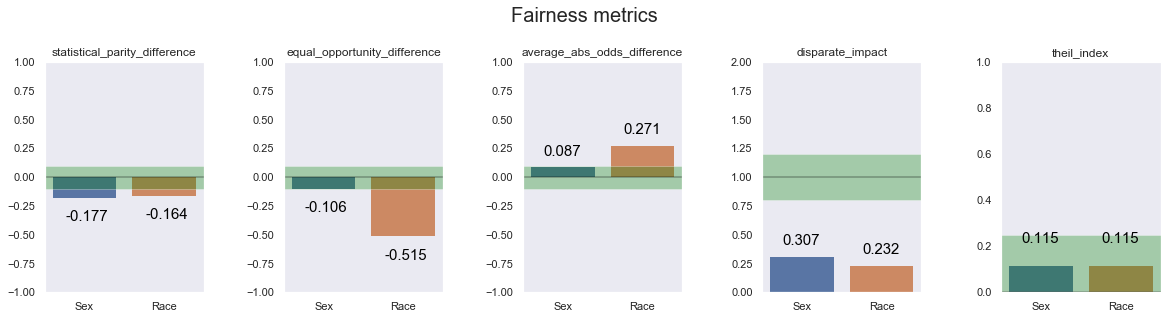In :
# This DataFrame is created to stock differents models and fair metrics that we produce in this notebook
algo_metrics = pd.DataFrame(columns=['model', 'fair_metrics', 'prediction', 'probs'])

def add_to_df_algo_metrics(algo_metrics, model, fair_metrics, preds, probs, name):
return algo_metrics.append(pd.DataFrame(data=[[model, fair_metrics, preds, probs]], columns=['model', 'fair_metrics', 'prediction', 'probs'], index=[name]))

In :
probs = rf.predict_proba(data_orig_test.features)
preds = rf.predict(data_orig_test.features)
algo_metrics = add_to_df_algo_metrics(algo_metrics, rf, fair, preds, probs, 'Origin')


As we can see, this first model is biased. The next step is to answer the question : How to fix it ?

### 3.2 How to fix it ?¶

AIF360 use 3 types of algorithms :

• Pre-processing algorithms : they are used before training the model
• In-processing algorithms : they are fair classifiers so it's during the training
• Post-processing algorithms : they are used after training the modelThis is the fairness pipeline. An example instantiation of this generic pipeline consists of loading data into a dataset object, transforming it into a fairer dataset using a fair pre-processing algorithm, learning a classifier from this transformed dataset, and obtaining predictions from this classifier. Metrics can be calculated on the original, transformed, and predicted datasets as well as between the transformed and predicted datasets. Many other instantiations are also possible (more information on the aif360 paper).

#### 3.2.1 Pre-processing algorithms ¶

There are 4 pre-processing algorithms but for 3 of them there is a problem : it will work only if the dataset have one protected attribute but here we have 2 : Sex and Race. But let's have a quick intro for all of them and at the end I will use the one that work for my case.

Because this notebook is an exploration of fairness into models I will only use 1 protected attribute : Sex, it will allow me to use almost all the algorithms (but for your information the Reweighing algo can works with all protected so that's why I will create a function that gives me the protected and unprotected attributes.

You can find all the code information on the documentation

In :
def get_attributes(data, only_one=True):
unprivileged_groups = []
privileged_groups = []

for attr in data.protected_attribute_names:
idx = data.protected_attribute_names.index(attr)
privileged_groups.append({attr:data.privileged_protected_attributes[idx]})
unprivileged_groups.append({attr:data.unprivileged_protected_attributes[idx]})
if only_one:
break

return privileged_groups, unprivileged_groups


##### 3.2.1.1 Disparate impact remover¶

Source : Feldman et al., 2015

Disparate impact remover is a preprocessing technique that edits feature values increase group fairness while preserving rank-ordering within groups. If you want to see how it works you can take a look on an example Notebook from the GitHub of AIF360.

Some code example :

from aif360.algorithms.preprocessing import DisparateImpactRemover
DIR = DisparateImpactRemover()
data_transf_train = DIR.fit_transform(data_orig_train)

##### 3.2.1.2 Learning fair representations¶

Source : Zemel et al., 2013

Learning fair representations is a pre-processing technique that finds a latent representation which encodes the data well but obfuscates information about protected attributes. You can find more information on the demo notebook.

In :
privileged_groups, unprivileged_groups = get_attributes(data_orig_train, only_one=True)

LFR = LFR(unprivileged_groups=unprivileged_groups, privileged_groups=privileged_groups, k=1, verbose=0)
# LFR.fit(data_orig_train)
data_transf_train = LFR.fit_transform(data_orig_train)

# Train and save the model
rf_transf = train_model(rf, data_transf_train)

data_transf_test = LFR.transform(data_orig_test)
fair = get_fair_metrics_and_plot(data_transf_test, rf_transf, plot=False)

# algo_metrics = add_to_df_algo_metrics(algo_metrics, rf_transf, fair, 'LFR')

250 41993.58928923252
500 33883.92930598489
750 33625.13761517554
1000 33625.135392161435
1250 33611.92929107428

WARNING:root:There are some instances in the dataset which are not designated as either privileged or unprivileged. Are you sure this is right?
WARNING:root:There are some instances in the dataset which are not designated as either privileged or unprivileged. Are you sure this is right?


##### 3.2.1.3 Optimized preprocessing¶

Source : Calmon et al., 2017

Optimized preprocessing is a preprocessing technique that learns a probabilistic transformation that edits the features and labels in the data with group fairness, individual distortion, and data fidelity constraints and objectives. There is also a demo notebook on the aif360 GitHub.

To be honest I tried to work with this one but it's more complicated : it uses options that you have to configure yourself and I don't really find how to choose it. Also it use an Optimizer and I didn't find how to build this class. (I didn't read the paper about this algorithm)

##### 3.2.1.4 Reweighing¶

Source : Kamiran and Calders, 2012

Reweighing is a preprocessing technique that Weights the examples in each (group, label) combination differently to ensure fairness before classification.

In :
privileged_groups, unprivileged_groups = get_attributes(data_orig_train, only_one=False)

RW = Reweighing(unprivileged_groups=unprivileged_groups, privileged_groups=privileged_groups)
# RW.fit(data_orig_train)
data_transf_train = RW.fit_transform(data_orig_train)

# Train and save the model
rf_transf = train_model(rf, data_transf_train)

data_transf_test = RW.transform(data_orig_test)
fair = get_fair_metrics_and_plot(data_orig_test, rf_transf, plot=False)
probs = rf_transf.predict_proba(data_orig_test.features)
preds = rf_transf.predict(data_orig_test.features)

algo_metrics = add_to_df_algo_metrics(algo_metrics, rf_transf, fair, preds, probs, 'Reweighing')

WARNING:root:There are some instances in the dataset which are not designated as either privileged or unprivileged. Are you sure this is right?
WARNING:root:There are some instances in the dataset which are not designated as either privileged or unprivileged. Are you sure this is right?


#### 3.2.2 In-processing algorithms¶

It exists 3 in-processing algorithms with aif360. But I will only use 2 : Adversarial Debiasing wich is using TensorFlow and Prejudice Remover Regularizer. I'm not using the last one : ART Classifier, because I didn't found any documentation that show how it works (maybe I didn't search enough 🙄).

You can find all the code information on the documentation

Source : Zhang et al., 2018

Adversarial debiasing is an in-processing technique that learns a classifier to maximize prediction accuracy and simultaneously reduce an adversary’s ability to determine the protected attribute from the predictions. This approach leads to a fair classifier as the predictions cannot carry any group discrimination information that the adversary can exploit.

In :
privileged_groups, unprivileged_groups = get_attributes(data_orig_train, only_one=True)

# sess.close()
# tf.reset_default_graph()
sess = tf.Session()

unprivileged_groups = unprivileged_groups,
scope_name='debiased_classifier',
num_epochs=42,
debias=True,
sess=sess)

debiased_model.fit(data_orig_train)

fair = get_fair_metrics_and_plot(data_orig_test, debiased_model, plot=False, model_aif=True)
data_pred = debiased_model.predict(data_orig_test)


epoch 0; iter: 0; batch classifier loss: 2.027651; batch adversarial loss: 0.696884
epoch 1; iter: 0; batch classifier loss: 0.535001; batch adversarial loss: 0.708483
epoch 2; iter: 0; batch classifier loss: 0.486652; batch adversarial loss: 0.671215
epoch 3; iter: 0; batch classifier loss: 0.452246; batch adversarial loss: 0.645171
epoch 4; iter: 0; batch classifier loss: 0.502617; batch adversarial loss: 0.609100
epoch 5; iter: 0; batch classifier loss: 0.423729; batch adversarial loss: 0.640453
epoch 6; iter: 0; batch classifier loss: 0.418263; batch adversarial loss: 0.621099
epoch 7; iter: 0; batch classifier loss: 0.382579; batch adversarial loss: 0.585946
epoch 8; iter: 0; batch classifier loss: 0.372982; batch adversarial loss: 0.592329
epoch 9; iter: 0; batch classifier loss: 0.341219; batch adversarial loss: 0.644888
epoch 10; iter: 0; batch classifier loss: 0.435262; batch adversarial loss: 0.581868
epoch 11; iter: 0; batch classifier loss: 0.435852; batch adversarial loss: 0.649803
epoch 12; iter: 0; batch classifier loss: 0.461696; batch adversarial loss: 0.640365
epoch 13; iter: 0; batch classifier loss: 0.493184; batch adversarial loss: 0.623321
epoch 14; iter: 0; batch classifier loss: 0.428212; batch adversarial loss: 0.655655
epoch 15; iter: 0; batch classifier loss: 0.349279; batch adversarial loss: 0.662475
epoch 16; iter: 0; batch classifier loss: 0.401383; batch adversarial loss: 0.642877
epoch 17; iter: 0; batch classifier loss: 0.375208; batch adversarial loss: 0.645407
epoch 18; iter: 0; batch classifier loss: 0.352355; batch adversarial loss: 0.653866
epoch 19; iter: 0; batch classifier loss: 0.381276; batch adversarial loss: 0.634348
epoch 20; iter: 0; batch classifier loss: 0.368791; batch adversarial loss: 0.568455
epoch 21; iter: 0; batch classifier loss: 0.362154; batch adversarial loss: 0.618681
epoch 22; iter: 0; batch classifier loss: 0.327374; batch adversarial loss: 0.627193
epoch 23; iter: 0; batch classifier loss: 0.417629; batch adversarial loss: 0.574505
epoch 24; iter: 0; batch classifier loss: 0.344165; batch adversarial loss: 0.621186
epoch 25; iter: 0; batch classifier loss: 0.335994; batch adversarial loss: 0.655801
epoch 26; iter: 0; batch classifier loss: 0.373382; batch adversarial loss: 0.586958
epoch 27; iter: 0; batch classifier loss: 0.376127; batch adversarial loss: 0.709685
epoch 28; iter: 0; batch classifier loss: 0.410763; batch adversarial loss: 0.635980
epoch 29; iter: 0; batch classifier loss: 0.472456; batch adversarial loss: 0.580156
epoch 30; iter: 0; batch classifier loss: 0.445524; batch adversarial loss: 0.585510
epoch 31; iter: 0; batch classifier loss: 0.466579; batch adversarial loss: 0.604531
epoch 32; iter: 0; batch classifier loss: 0.365391; batch adversarial loss: 0.645607
epoch 33; iter: 0; batch classifier loss: 0.358953; batch adversarial loss: 0.573522
epoch 34; iter: 0; batch classifier loss: 0.467547; batch adversarial loss: 0.612585
epoch 35; iter: 0; batch classifier loss: 0.467910; batch adversarial loss: 0.556397
epoch 36; iter: 0; batch classifier loss: 0.329569; batch adversarial loss: 0.585394
epoch 37; iter: 0; batch classifier loss: 0.429180; batch adversarial loss: 0.615465
epoch 38; iter: 0; batch classifier loss: 0.363523; batch adversarial loss: 0.616181
epoch 39; iter: 0; batch classifier loss: 0.309973; batch adversarial loss: 0.591368
epoch 40; iter: 0; batch classifier loss: 0.395164; batch adversarial loss: 0.624585
epoch 41; iter: 0; batch classifier loss: 0.405081; batch adversarial loss: 0.610189

WARNING:root:There are some instances in the dataset which are not designated as either privileged or unprivileged. Are you sure this is right?
WARNING:root:There are some instances in the dataset which are not designated as either privileged or unprivileged. Are you sure this is right?


##### 3.2.2.2 Prejudice Remover Regularizer¶

Source : Kamishima et al., 2012

Prejudice remover is an in-processing technique that adds a discrimination-aware regularization term to the learning objective.

In :
debiased_model = PrejudiceRemover(sensitive_attr="Sex", eta = 25.0)
debiased_model.fit(data_orig_train)

fair = get_fair_metrics_and_plot(data_orig_test, debiased_model, plot=False, model_aif=True)
data_pred = debiased_model.predict(data_orig_test)

algo_metrics = add_to_df_algo_metrics(algo_metrics, debiased_model, fair, data_pred.labels, data_pred.scores, 'PrejudiceRemover')

WARNING:root:There are some instances in the dataset which are not designated as either privileged or unprivileged. Are you sure this is right?
WARNING:root:There are some instances in the dataset which are not designated as either privileged or unprivileged. Are you sure this is right?


#### 3.2.3 Post-processing algorithms¶

It exists 3 post-processing algorithms with aif360.

You can find all the code information on the documentation

I prepare the predict dataset for all the algorithms that require a dataset with predict scores and labels.

In :
data_orig_test_pred = data_orig_test.copy(deepcopy=True)

# Prediction with the original RandomForest model
scores = np.zeros_like(data_orig_test.labels)
scores = rf.predict_proba(data_orig_test.features)[:,1].reshape(-1,1)
data_orig_test_pred.scores = scores

preds = np.zeros_like(data_orig_test.labels)
preds = rf.predict(data_orig_test.features).reshape(-1,1)
data_orig_test_pred.labels = preds

def format_probs(probs1):
probs1 = np.array(probs1)
probs0 = np.array(1-probs1)
return np.concatenate((probs0, probs1), axis=1)


##### 3.2.3.1 Calibrated Equality of Odds¶

Source : Pleiss et al., 2017

Calibrated equalized odds postprocessing is a post-processing technique that optimizes over calibrated classifier score outputs to find probabilities with which to change output labels with an equalized odds objective.

In :
privileged_groups, unprivileged_groups = get_attributes(data_orig_train, only_one=True)

cost_constraint = "fnr" # "fnr", "fpr", "weighted"

CPP = CalibratedEqOddsPostprocessing(privileged_groups = privileged_groups,
unprivileged_groups = unprivileged_groups,
cost_constraint=cost_constraint,
seed=42)

CPP = CPP.fit(data_orig_test, data_orig_test_pred)
data_transf_test_pred = CPP.predict(data_orig_test_pred)

fair = fair_metrics(data_orig_test, data_orig_test_pred, pred_is_dataset=True)

CPP,
fair,
data_transf_test_pred.labels,
format_probs(data_transf_test_pred.scores),
'CalibratedEqOdds')

WARNING:root:There are some instances in the dataset which are not designated as either privileged or unprivileged. Are you sure this is right?
WARNING:root:There are some instances in the dataset which are not designated as either privileged or unprivileged. Are you sure this is right?


##### 3.2.3.2 Equality of Odds¶

Source : Hardt et al., 2016

Equalized odds postprocessing is a post-processing technique that solves a linear program to find probabilities with which to change output labels to optimize equalized odds.

In :
privileged_groups, unprivileged_groups = get_attributes(data_orig_train, only_one=True)

EOPP = EqOddsPostprocessing(privileged_groups = privileged_groups,
unprivileged_groups = unprivileged_groups,
seed=42)

EOPP = EOPP.fit(data_orig_test, data_orig_test_pred)
data_transf_test_pred = EOPP.predict(data_orig_test_pred)

fair = fair_metrics(data_orig_test, data_transf_test_pred, pred_is_dataset=True)

EOPP,
fair,
data_transf_test_pred.labels,
format_probs(data_transf_test_pred.scores),
'EqOdds')

WARNING:root:There are some instances in the dataset which are not designated as either privileged or unprivileged. Are you sure this is right?
WARNING:root:There are some instances in the dataset which are not designated as either privileged or unprivileged. Are you sure this is right?


##### 3.2.3.3 Reject Option Classification¶

Source : Kamishima et al., 2012

Reject option classification is a postprocessing technique that gives favorable outcomes to unpriviliged groups and unfavorable outcomes to priviliged groups in a confidence band around the decision boundary with the highest uncertainty.

In :
privileged_groups, unprivileged_groups = get_attributes(data_orig_train, only_one=True)

ROC = RejectOptionClassification(privileged_groups = privileged_groups,
unprivileged_groups = unprivileged_groups)

ROC = ROC.fit(data_orig_test, data_orig_test_pred)
data_transf_test_pred = ROC.predict(data_orig_test_pred)

fair = fair_metrics(data_orig_test, data_transf_test_pred, pred_is_dataset=True)

ROC,
fair,
data_transf_test_pred.labels,
format_probs(data_transf_test_pred.scores),
'RejectOption')

WARNING:root:There are some instances in the dataset which are not designated as either privileged or unprivileged. Are you sure this is right?
WARNING:root:There are some instances in the dataset which are not designated as either privileged or unprivileged. Are you sure this is right?


### 3.3 Compare all the algorithms for one attribute¶

In :
def compare_fair_metrics(algo_metrics, attr='Sex'):

df_metrics = pd.DataFrame(columns=algo_metrics.loc['Origin','fair_metrics'].columns.values)
for fair in algo_metrics.loc[:,'fair_metrics']:
df_metrics = df_metrics.append(fair.loc[attr], ignore_index=True)

df_metrics.index = algo_metrics.index.values

display(df_metrics)
plot_fair_metrics_plotly(df_metrics)
score = score_fair_metrics(df_metrics)
plot_score_fair_metrics(score)

In :
compare_fair_metrics(algo_metrics)

statistical_parity_difference equal_opportunity_difference average_abs_odds_difference disparate_impact theil_index
Origin -0.177133 -0.105927 0.087368 0.307059 0.115040
Reweighing -0.178060 -0.098609 0.085025 0.303432 0.115235
AdvDebiasing 0.003788 0.350229 0.183090 1.030742 0.182728
PrejudiceRemover -0.163178 -0.262306 0.173923 0.088076 0.190125
CalibratedEqOdds -0.178060 -0.098609 0.085025 0.303432 0.115235
EqOdds -0.024443 0.134688 0.085385 0.861981 0.162305
RejectOption -0.046664 0.219760 0.114301 0.715088 0.144189
score nb_valid
EqOdds 0.544840 4.0
Reweighing 1.173497 3.0
CalibratedEqOdds 1.173497 3.0
RejectOption 0.809826 2.0
Origin 1.178410 2.0
PrejudiceRemover 1.701454 1.0

### 3.4 Find the fairest algorithm¶

In :
final = score_all_attr(algo_metrics)
plot_score_fair_metrics(final)

score nb_valid
EqOdds 1.767792 5.0
Reweighing 2.838542 4.0
CalibratedEqOdds 2.838542 4.0
RejectOption 2.164320 3.0
PrejudiceRemover 2.861810 3.0
Origin 3.012171 3.0

So now we see that it's the EqOdds algorithm that gives us the fairest model but it's not completly fair only 6 metrics for 10 valid the fair of the model, mostly for the Race column because we didn't unbias the column, but for the Sex column we can see that 4 metrics valid fair in the model so it's a great improvment ! 👍

Now let's compare our algorithm for the performance.

### 3.5 Compare algorithms performance¶

In :
plot_compare_model_performance(algo_metrics, data_orig_test)

Accuracy F1 Score
Origin 0.860682 0.683562
Reweighing 0.860579 0.683108
CalibratedEqOdds 0.860579 0.683108
RejectOption 0.847067 0.609514
EqOdds 0.812059 0.544191
PrejudiceRemover 0.800082 0.457048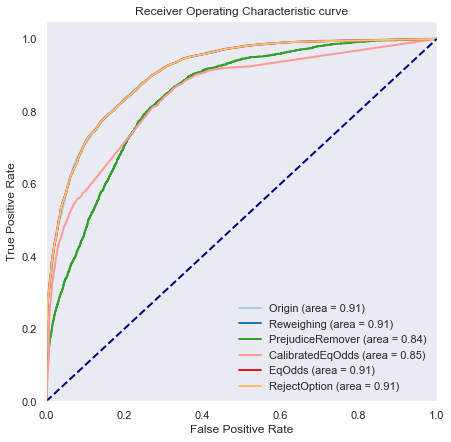We can see that the original model still has a great accuracy and F1 Score. I remind you that here I didn't optimize the models to be excellent I used default configurations.

Now you have to ask yourself the question, what is more important : performance or fair ?

## 4. Interpretable model¶

### 4.1 Feature importance¶

The question of model interpretation is becoming really important and the most common way to explain a model is to use feature importance. It's usefull to get an insight of what the model is using in feature to predict something but it has limits. First of all let's see how it works.

Feature importance changes according to the model that we're using. It's important to know that it exists differents ways to measure feature importance according to the model so I will introduce some measure for 3 types of models : Linear Regression, Decision Tree and Tree models.

#### 4.1.1 Linear Regression¶

For the Linear Regression if we have standardized the features the coefficient represents the influence of the feature on the model. So for a Linear model with a formula :

$$h_\theta(X) = \theta_0x_0 + \theta_1x_1 + ... + + \theta_nx_n = \theta^TX$$

We can just take all the theta with the vector $\theta$ and it's our importance for each feature.

#### 4.1.2 Decision Tree¶

For the Decision Tree, feature importance is not really important because this model is human interpretable. For a prediction you just have to follow the leaves.

#### 4.1.3 Tree models (e.g Random Forest)¶

I think this type of models (Random Forest, XGBoost, LGBM, ...) is well known in the industry today so the feature importance of those models are logically the most used for classification. So the most used measure of feature importance for Tree models is Gini Importance or Mean Decrease in Impurity (MDI).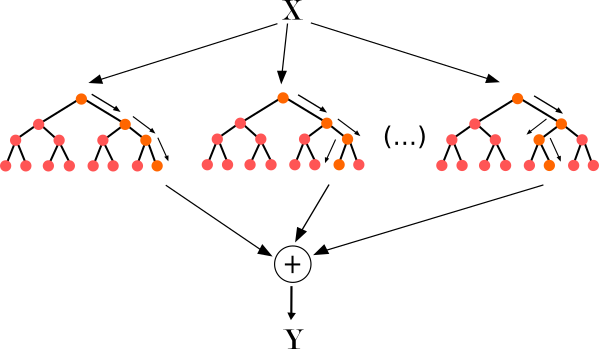MDI counts the times a feature is used to split a node, weighted by the number of samples it splits.

We can calculate it with this formula :

$$Imp(X_j)=\frac{1}{M}\sum_{m=1}^{M}\sum_{t\in \varphi_m} (j_t=j)[p(t)\Delta i(t)]$$

Here we calculate the importance of variable $X_j$ for an ensemble of $M$ trees. $j_t$ denotes the variable used at node $t$, $p(t) = \frac{N_t}{N}$ is the proportion of rows used in that node (if it's the first node it will be 1) and $\Delta i(t)$ is the impurity reduction at node $t$ : we can use Gini index or Entropy to measure impurity.

I find out this formula from this course : Understanding variable importances in forests of randomized trees

Also if you want more informations about others Tree models measure of feature importance you can take a look at this publication : Feature Importance Measures for Tree Models — Part I

Now let's see what is the feature importance of our Random Forest model.

In :
rf.feature_importances_
feature_imp = pd.Series(rf.feature_importances_, index=data_orig_train.feature_names)
feature_imp.sort_values(ascending=False, inplace=True)

In :
plt.figure(figsize=(10,7))
ax = sns.barplot(y=feature_imp.index.values, x=feature_imp,orient='h')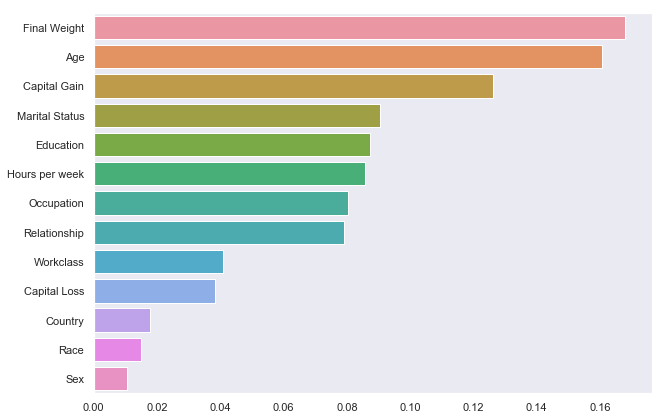We see that Final Weight, Age and Capital Gain are the features that are the most importance for this model if we use feature importance from sklearn RandomForestClassifier. But now we arrive at the limits of feature importance, if I want to know wich feature are important on a specific prediction I can't know that I just can suppose that it follows the feature importance measure but I can not be sure.

### 4.2 LIME¶

Source : LIME GitHub repository

So with the objective to find out wich features are important for a specific prediction appear LIME : Local interpretable model-agnostic explanations it means that LIME can explain any models in Machine Learning. We can think it like the model is a black box that just have the input and output to find out what feature is important.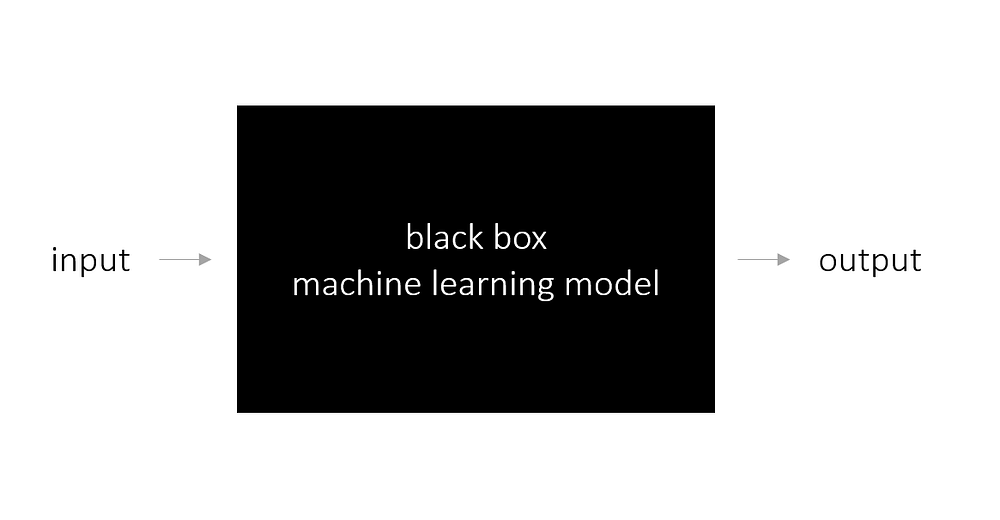To understand how it works we have to define it is important to distinguish between features and interpretable data representations. As mentioned before, interpretable explanations need to use a representation that is understandable to humans, regardless of the actual features used by the model. For example, a possible interpretable representation for text classification is a binary vector indicating the presence or absence of a word.

Now Let $f$ be the original prediction model to be explained and $g$ the explanation model such as a linear regression model. Here, we focus on local methods designed to explain a prediction $f(x)$ based on a single input $x$. Explanation models often use simplified inputs $x$ that map to the original inputs through a mapping function $x = h_x(x')$. Local methods try to ensure $g(z') ≈ f(h_x(z'))$ whenever $z' ≈ x'$ so it's a perturbed sample (Note that $hx(x') = x$ even though x may contain less information than $x$ because $h_x$ is specific to the current input $x'$).

The explanation model that is a linear function of binary variables that follows this formula : $$g(z') = \phi_0 + \sum_{i=1}^{M}\phi_i z_i'$$

where $z' \in \{0, 1\}^M$, $M$ is the number of simplified input features, and $\phi_i \in R$

To find $\phi$, LIME minimizes the following objective function : $$\xi = \underset{g \in G}{arg min} \space L(f,g,\pi_{x'}) + \Omega(g)$$

Faithfulness of the explanation model $g(z')$ to the original model $f(h_x(z'))$ is enforced through the loss $L$ over a set of samples in the simplified input space weighted by the local kernel $\pi_{x'}$ (which calculate the distance between the perturbed sample and the original point). $\Omega$ penalizes the complexity of $g$. Since in LIME $g$ follows the linear equation above and $L$ is a squared loss, this equation can be solved using penalized linear regression.

So at the end we can obtain a explanation model like this :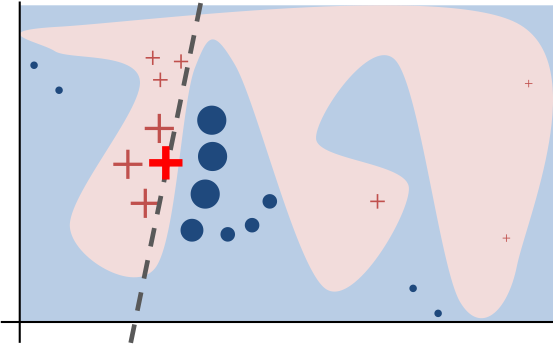Now let's use LIME on our dataset and original model (Random Forest).

In :
# Convert AIF360 StandardDataset into a pandas DataFrame
test_interp = pd.DataFrame(data=data_orig_test.features,
columns=data_orig_test.feature_names)

categorical_features = ["Workclass", "Education", "Marital Status","Occupation", "Relationship", "Race", "Sex", "Country"]
categorical_features_idx = [np.where(test_interp.columns.values == col) for col in categorical_features]
categorical_names_LIME = {}

for feature, idx in zip(categorical_features, categorical_features_idx):
categorical_names_LIME[idx] = categorical_names[feature]

# Reverse the MinMaxScaler to get the original values back.
for feat in test_interp.columns.values:
if feat in numerical_features:
test_interp[feat] = encoders[feat].inverse_transform(np.array(test_interp[feat]).reshape(-1, 1))

In :
# Initiate the LIME explainer
explainer = lime.lime_tabular.LimeTabularExplainer(test_interp.values,
feature_names=test_interp.columns.values,
class_names=['Income <= 50K', 'Income > 50K'],